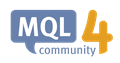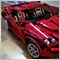# combined two indicators compiled but no result60

Hello All,

I have two codes one Doda stochastic and EMA cross over alert, i have combined both. ( i have set correctly i guess the buffer values, setindex and all but because of two "for loops" i am stuck and two counter in same code), I have modified code as much as i could. (compiled when counter name change)

(Note: xx are Values)

my conditions  when

Buy Alert:

(doda stochastic above xx) && (ema slow  xx above ema fast xx) = BUY alert

Ex:

`// if((ExtHistoBuffer <indicator_level1 && BuyAlert==False) && (CrossUp[i] > CrossDown[i] && alertTag!=Time) )`

Sell Alert:

(doda stochastic below xx) && (ema slow  xx below ema fast xx) = sell alert

Ex:

`// if((ExtHistoBuffer >indicator_level2 && BuyAlert==False) && (CrossUp[i] < CrossDown[i] && alertTag!=Time) )`

```//+------------------------------------------------------------------+
//|                                         EMA-Crossoverand DODA_Signal.mq4 |
//|                  |
//+------------------------------------------------------------------+

/*
+------------------------------------------------------------------+
TEST            |
+------------------------------------------------------------------+
*/
#include <stdlib.mqh>

#property indicator_separate_window
#property indicator_minimum 0.00
#property indicator_maximum 100.00
#property indicator_color1 Lime
#property indicator_color2 Red
#property indicator_color3 SeaGreen
#property indicator_color4 Red
#property indicator_buffers 4

#property indicator_level1 5
#property indicator_level2 95

//+------------------------------------------------------------------+
//| Common External variables                                        |
//+------------------------------------------------------------------+
//+------------------------------------------------------------------+
//| Special Convertion Functions                                     |
//+------------------------------------------------------------------+

int LastTradeTime;
double CrossUp[];
double CrossDown[];
double ExtHistoBuffer[];
double ExtHistoBuffer2[];
bool BuyAlert=false,SellAlert=false;
//+------------------------------------------------------------------+
//+------------------------------------------------------------------+
//| External variables                                               |
//+------------------------------------------------------------------+
extern double Slw = 8;
extern double Pds = 13;
extern double Slwsignal= 9;
extern int    Barcount = 2000;
extern int FasterEMA = 4;
extern int SlowerEMA = 8;
extern bool SoundON=False;

double alertTag;
double control=2147483647;

//|                                                                  |
//+------------------------------------------------------------------+
void SetLoopCount(int loops)
{
}
//+------------------------------------------------------------------+
//|                                                                  |
//+------------------------------------------------------------------+
void SetIndexValue(int shift,double value)
{
ExtHistoBuffer[shift]=value;
//  Print ("ExtHistoBuffer :" ,value);    // green
}
//+------------------------------------------------------------------+
//|                                                                  |
//+------------------------------------------------------------------+
void SetIndexValue2(int shift,double value)
{
ExtHistoBuffer2[shift]=value;
//  Print ("ExtHistoBuffer2 :" ,value);    // green
}
//+------------------------------------------------------------------+
//|                                                                  |
//+------------------------------------------------------------------+
double GetIndexValue(int shift)
{
return(ExtHistoBuffer[shift]);
}
//+------------------------------------------------------------------+
//|                                                                  |
//+------------------------------------------------------------------+
double GetIndexValue2(int shift)
{
return(ExtHistoBuffer2[shift]);
}
//+------------------------------------------------------------------+
//| End                                                              |
//+------------------------------------------------------------------+

//+------------------------------------------------------------------+
//| Initialization                                                   |
//+------------------------------------------------------------------+

//+------------------------------------------------------------------+
//| Custom indicator initialization function                         |
//+------------------------------------------------------------------+
int init()
{
//---- indicators
SetIndexStyle(0, DRAW_ARROW, EMPTY,3);
SetIndexArrow(0, 233);
SetIndexBuffer(0, CrossUp);
SetIndexStyle(1, DRAW_ARROW, EMPTY,3);
SetIndexArrow(1, 234);
SetIndexBuffer(1, CrossDown);
SetIndexStyle(2,DRAW_LINE,STYLE_SOLID);
SetIndexBuffer(2,ExtHistoBuffer);
SetIndexStyle(3,DRAW_LINE,STYLE_SOLID);
SetIndexBuffer(3,ExtHistoBuffer2);
return(0);

}
//+------------------------------------------------------------------+
//| Custom indicator deinitialization function                       |
//+------------------------------------------------------------------+
int deinit()
{
//----

//----
return(0);
}
//+------------------------------------------------------------------+
//| Custom indicator iteration function                              |
//+------------------------------------------------------------------+
int start() {

//+------------------------------------------------------------------+
//| Local variables                                                  |
//+------------------------------------------------------------------+
double AA = 0;
double bb = 0;
double aa1= 0;
double cnt1=0;
int shift=0;
double cnt=0;
double loopbegin=0;
double loopbegin2 = 0;
double loopbegin3 = 0;
bool first=True;
double prevbars=0;
double sum=0;
double smconst=0;
double smconst1=0;
double prev=0;
double prev1 = 0;
double prev2 = 0;
double prev3 = 0;
double weight = 0;
double linear = 0;
double MAValue= 0;
double MAValue2 = 0;
double mavalue3 = 0;
string MAstring = "";
double MyHigh= 0;
double MyLow = 0;
int counter1=0;
double Price=0;
double Price1=0;
double tmpDevAA=0;

SetLoopCount(0);
smconst=2/(1+Slw);
smconst1=2/(1+Slwsignal);

int limit;
int i;
int counter;
double fasterEMAnow, slowerEMAnow, fasterEMAprevious, slowerEMAprevious, fasterEMAafter, slowerEMAafter;
double Range, AvgRange;
//Function start

int counted_bars=IndicatorCounted();

if (Bars<Barcount) return(-1);

loopbegin = loopbegin+1;
for(shift =Barcount-1;shift >=0;shift --)
{
prev=GetIndexValue2(shift+1);
// Yousky 15/05/2006 - Change to avoid Zero divide exception.
AA=0;
tmpDevAA=(High[Highest(NULL,0,MODE_HIGH,shift+Pds,Pds)]-Low[Lowest(NULL,0,MODE_LOW,shift+Pds,Pds)]);

if(tmpDevAA!=0)
AA=100*((Close[shift]-Low[Lowest(NULL,0,MODE_LOW,shift+Pds,Pds)])/tmpDevAA);
// ---
MAValue2=smconst *(AA-prev)+prev;
SetIndexValue2(shift,MAValue2);
loopbegin=loopbegin-1;
}

loopbegin2=loopbegin2+1;
for(shift=Barcount-Pds;shift>=0;shift --)
{
MyHigh= -999999;
MyLow = 99999999;
for(counter1=shift;counter1<=Pds+shift;counter1++)
{
Price=GetIndexValue2(counter1);
if(Price>MyHigh)
MyHigh=Price;
if(Pds<=0)
MyHigh=Price;
if(Price<MyLow)
MyLow=Price;
if(Pds<=0)
MyLow=Price;
}

prev1=GetIndexValue(shift+1);
aa1=GetIndexValue2(shift);

// Yousky 15/05/2006 - Change to avoid Zero divide exception.
bb=0;
if((MyHigh-MyLow)!=0)
bb=100*(aa1-MyLow)/(MyHigh-MyLow);
// ---
MAValue=smconst *(bb-prev1)+prev1;
SetIndexValue(shift,MAValue);
loopbegin2=loopbegin2-1;
}

//Print (MAValue);  // green

loopbegin3=loopbegin3+1;
for(shift=Barcount;shift>=0;shift --)
{
prev2=GetIndexValue2(shift+1);
prev3=GetIndexValue(shift);
mavalue3=smconst1 *(prev3-prev2)+prev2;

SetIndexValue2(shift,mavalue3);
loopbegin3=loopbegin3-1;
}

//I want something like this below commented:
if((ExtHistoBuffer <indicator_level1 && BuyAlert==False) && (CrossUp[i] > CrossDown[i] && alertTag!=Time) )

//if(ExtHistoBuffer<indicator_level1 && BuyAlert==False)
{ Alert("Buy ",Symbol());
BuyAlert=True;
SellAlert=False;
}
if((ExtHistoBuffer >indicator_level2 && BuyAlert==False) && (CrossUp[i] < CrossDown[i] && alertTag!=Time) )

//  if(ExtHistoBuffer>indicator_level2 && SellAlert==False)
{
// sell signal
Alert("Sell ",Symbol());
BuyAlert=false;
SellAlert=True;
}

//+------------------------------------------------------------------+
//| DODA Stochastic Code End                      |
//+------------------------------------------------------------------+

//+------------------------------------------------------------------+
//| EMA Code Start                     |
//+------------------------------------------------------------------+

//---- check for possible errors
if(counted_bars<0) return(-1);
//---- last counted bar will be recounted
if(counted_bars>0) counted_bars--;

limit=Bars-counted_bars;

for(i = 0; i <= limit; i++) {

counter=i;
Range=0;
AvgRange=0;
for (counter=i ;counter<=i+9;counter++)
{
AvgRange=AvgRange+MathAbs(High[counter]-Low[counter]);
}
Range=AvgRange/10;

fasterEMAnow = iMA(NULL, 0, FasterEMA, 0, MODE_EMA, PRICE_CLOSE, i);
fasterEMAprevious = iMA(NULL, 0, FasterEMA, 0, MODE_EMA, PRICE_CLOSE, i+1);
fasterEMAafter = iMA(NULL, 0, FasterEMA, 0, MODE_EMA, PRICE_CLOSE, i-1);

slowerEMAnow = iMA(NULL, 0, SlowerEMA, 0, MODE_EMA, PRICE_CLOSE, i);
slowerEMAprevious = iMA(NULL, 0, SlowerEMA, 0, MODE_EMA, PRICE_CLOSE, i+1);
slowerEMAafter = iMA(NULL, 0, SlowerEMA, 0, MODE_EMA, PRICE_CLOSE, i-1);

if ((fasterEMAnow > slowerEMAnow) && (fasterEMAprevious < slowerEMAprevious) && (fasterEMAafter > slowerEMAafter)) {
CrossUp[i] = Low[i] - Range*0.5;
}
else if ((fasterEMAnow < slowerEMAnow) && (fasterEMAprevious > slowerEMAprevious) && (fasterEMAafter < slowerEMAafter)) {
CrossDown[i] = High[i] + Range*0.5;
}

//Not Required Below Code
/*     if (SoundON==true && i==1 && CrossUp[i] > CrossDown[i] && alertTag!=Time){
Alert("EMA Cross Trend going Down on ",Symbol()," ",Period());
alertTag = Time;
}
if (SoundON==true && i==1 && CrossUp[i] < CrossDown[i] && alertTag!=Time){
Alert("EMA Cross Trend going Up on ",Symbol()," ",Period());
alertTag = Time;
} */
//+------------------------------------------------------------------+
//| EMA Code Start                     |
//+------------------------------------------------------------------+

}
return(0);
}

```

Please let me know where i am wrong.

Thankyou.

Files:60

looks like i should call this https://docs.mql4.com/indicators/ima instead of  combining two separate indicator.

Any idea? Anyone?iMA - Technical Indicators - MQL4 Reference
• docs.mql4.com
iMA - Technical Indicators - MQL4 Reference1014

samvendor:

I have two codes one Doda stochastic and EMA cross over alert, i have combined both. ( i have set correctly i guess the buffer values, setindex and all but because of two "for loops" i am stuck and two counter in same code), I have modified code as much as i could. (compiled when counter name change)

(Note: xx are Values)

my conditions  when

Buy Alert:

(doda stochastic above xx) && (ema slow  xx above ema fast xx) = BUY alert

Ex:

Sell Alert:

(doda stochastic below xx) && (ema slow  xx below ema fast xx) = sell alert

Ex:

Please let me know where i am wrong.

You need to be more specific - what do you mean by "no result"?

When I run your code, I can see two lines and arrows for both directions plotted within the same indicator sub-window. Only problem is your arrow positions are wrong.60

Seng Joo Thio:

You need to be more specific - what do you mean by "no result"?

When I run your code, I can see two lines and arrows for both directions plotted within the same indicator sub-window. Only problem is your arrow positions are wrong.

But in my sub window there is nothing its all blank1014

samvendor:
But in my sub window there is nothing its all blank

Probably due to this line:

``` if (Bars<Barcount) return(-1);
```
Change your Barcount to a smaller value, or go to Tools->Options->Charts and increase the two Max bars (both in history and in chart) to beyond Barcount.60

Seng Joo Thio:

Probably due to this line:

Change your Barcount to a smaller value, or go to Tools->Options->Charts and increase the two Max bars (both in history and in chart) to beyond Barcount.

Hey Seng Thankyou, you were right it was because of the barcount, i will try to fix the arrow position.

To add comments, please log in or register